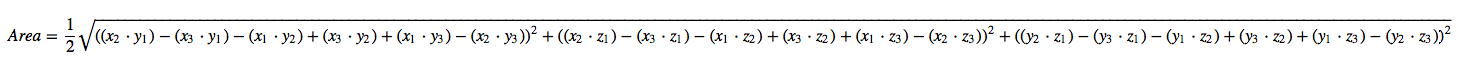# Area of Triangle in 3D

## Heron's Formula

### Area = Sqrt{ s * (s - a) * (s - b) * (s - c) }

Figure out the length of the sides of the triangle - (a, b, c)

a = Sqrt{(x1 - x2)^2 + (y1 - y2)^2 + (z1 - z2)^2}

b = Sqrt{(x1 - x3)^2 + (y1 - y3)^2 + (z1 - z3)^2}

c = Sqrt{(x2 - x3)^2 + (y2 - y3)^2 + (z2 - z3)^2}

s is the semiperimeter of the triangle; s = (a + b + b) / 2

Plug everything into Heron's formula to get your answer.

#### There is not much of a problem with this approach, but:

• If triangles are fine slivers, this algorithm for Heron's formula can be problematic.
• It is somewhat inefficient to calculate square roots on a computer. This algorithm requires the calculation of a square root four times, one for each of the sides and then within the formula itself. The question arises can this be done more efficiently if your use case requires thousands of triangle area calculations and you have no other use for the lengths of the triangles sides.

## Cross-Product

### Area = Sqrt{ (x2*y1 - x3*y1 - x1*y2 + x3*y2 + x1*y3 - x2*y3)^2 + (x2*z1 - x3*z1 - x1*z2 + x3*z2 + x1*z3 - x2*z3)^2 + (y2*z1 - y3*z1 - y1*z2 + y3*z2 + y1*z3 - y2*z3)^2 } / 2

You can learn about the cross-product approach by Googling the subject. I am not going to provide any background on this. Suffice to say, the area of a triangle in 3-D is equal to 1/2 the cross product of two vectors that represent any two sides of the triangle. If you do Google the subject you are likely to be shown matrices and calculations derived from those matrices which allow you to get the answer. I have put up this site for those who really do not want to know how the cross product is calculated and for whom matrices are not second nature. They are just looking for a formula to find the area of a triangle in 3_D that is more efficient than Heron's formula.

The formula is long and would be hard to remember, but it is easy for a computer and relatively efficient because it only requires the calculation of one square root.

If your screen is wide enough, you can see it formatted more clearly below